﻿ Dynamic Modeling of PGT using Analytical & Numerical Approach

### Dynamic Modeling of PGT using Analytical & Numerical Approach

S. S. Ghorpade, A. B. Kadam, D.A. Mane, S. H. Gawande, S. N. Shaikh

Journal of Mechanical Design and Vibration

## Dynamic Modeling of PGT using Analytical & Numerical Approach

S. S. Ghorpade1,, A. B. Kadam1, D.A. Mane1, S. H. Gawande1, S. N. Shaikh1

1Department of Mechanical Engineering, M. E. Society’s College of Engineering, Pune, S.P. Pune University, Maharashtra, India

### Abstract

Gears are one of the most critical components in industrial rotating machinery. There is a vast amount of literature on gear modelling. The objectives in dynamic modelling of gears has varied from vibration analysis and noise control, to transmissions errors and stability analysis over at least the past five decades. The ultimate goal of this paper is to perform planetary gear train modeling as in  to study the effect deflection and stresses on surface pitting and scoring. This paper is an extension of the work performed by the authors as in , in which the experimental work was carried out to study the effect of planet phasing on noise and subsequent resulting vibrations of Nylon-6 planetary gear drive.

• S. S. Ghorpade, A. B. Kadam, D.A. Mane, S. H. Gawande, S. N. Shaikh. Dynamic Modeling of PGT using Analytical & Numerical Approach. Journal of Mechanical Design and Vibration. Vol. 3, No. 1, 2015, pp 24-30. http://pubs.sciepub.com/jmdv/3/1/3
• Ghorpade, S. S., et al. "Dynamic Modeling of PGT using Analytical & Numerical Approach." Journal of Mechanical Design and Vibration 3.1 (2015): 24-30.
• Ghorpade, S. S. , Kadam, A. B. , Mane, D. , Gawande, S. H. , & Shaikh, S. N. (2015). Dynamic Modeling of PGT using Analytical & Numerical Approach. Journal of Mechanical Design and Vibration, 3(1), 24-30.
• Ghorpade, S. S., A. B. Kadam, D.A. Mane, S. H. Gawande, and S. N. Shaikh. "Dynamic Modeling of PGT using Analytical & Numerical Approach." Journal of Mechanical Design and Vibration 3, no. 1 (2015): 24-30.

 Import into BibTeX Import into EndNote Import into RefMan Import into RefWorks

12
Prev Next

### 1. Introduction

Planetary gears are essential parts of many precision power transmitting elements such as an automobile, tractors, wind turbines, helicopters, and aircraft engines, where high torque to weight ratios, large speed reductions in compact volumes, co-axial shaft arrangements, high reliability and superior efficiency are required. Gear stresses & vibrations are primary concerns in most planetary gear transmission applications, where the manifest problem may be noise or dynamic forces. Despite infinite advantages, the dynamics & noise induced by the vibration of planetary gear systems remains a key concern. Planetary gears have received considerably less research attention than single mesh gear pairs. There is a particular scarcity of analysis of two planetary gear systems & their dynamic response. Planet phasing provides an effective means to reduce planetary gear vibration . A significant advantage is its cost-effectiveness as no additional manufacturing processes & measuring instruments are needed. This paper focus on the study of two PGTs with different phasing (angular positions) while keeping every individual set unchanged. This paper focus on the study of dynamics of planetary gear set by using Analytical & Finite Element Methods. Large dynamic forces increase the risk of gear tooth or bearing failure. Kahraman and Blankenship [2, 3] performed experiments on a spur gear pair and observed various nonlinear phenomena including gear tooth contact loss, period-doubling and chaos. Tooth separations at large vibrations, which are common in spur–gear pairs, occur even in planetary gears as evident from the experiments by Botman . Planetary gear researchers have developed lumped-parameter models and deformable gear models to analyze gear dynamics . The literature mainly addresses static analysis, natural frequencies and vibration modes, modeling to estimate dynamic forces and responses, and cancellation of mesh forces using the planetary gear symmetry through mesh phasing. Studies by Cunliffe et al. , Botman , Hidaka and Terauchi , Hidaka et al. , and Kahraman [9–11] involve planetary gear models to estimate natural frequencies, vibration modes and dynamic forces. Lin and Parker [12, 13][12, 13] present a 2D rotational–translational degree of freedom spur gear model and mathematically show the unique modal properties of equally spaced and diametrically opposed planet systems. All modes can be classified as one of rotational, translational, or planet modes. The sensitivity of natural frequencies and modes to operating speeds and various design parameters are studied by Lin and Parker , who also examine natural frequency veering phenomena . Mesh stiffness-induced parametric instability is studied by Lin and Parker . A helical planetary gear model is formulated and the effect of mesh phasing on the dynamics of equally spaced planet systems is investigated by Kahraman  and Kahraman and Blankenship . In recent years, some researchers have used deformable gear body dynamic models. A unique finite element contact analysis program is used by Parker et al.  to model nonlinear spur gear dynamics. The finite element results compare favorably with experiments. Parker et al.  used the same finite element method to examine planetary gear dynamics. Kahraman and Vijayakar  studied the effect of ring gear flexibility on the static response of planetary gears using the finite element method. A recent study by Kahraman et al.  dynamically analyzes a planetary gear with thin rim using the same finite element method. Kahraman et al.  employed this finite element model to study tooth wear and its impact on the dynamic behavior of a planetary gear. These studies use the same commercial finite element tool , which is also adopted in this study.

Accurate analytical modeling, including proper mesh phasing relations and detailed characterization of the nonlinear dynamics of planetary gears, is needed to estimate relative gear noise and predict dynamic forces in industrial applications. Little work has been done to characterize the nonlinear effects of tooth separation on planetary gear dynamics. The scarcity of experimental studies  to understand the complex dynamics of planetary gears and the availability of finite element software specialized for gear dynamics motivated the present study. The objectives of this study are to: (a) characterize the complex, nonlinear dynamics of spur planetary gear systems using a unique finite element model as demonstrated in  and (b) propose a lumped-parameter analytical model that is validated by comparisons with the finite element results across the range of complicated nonlinear dynamics occurring in the system.

### 2. Experimental Set up

The main objective of this paper is to perform dynamic analysis of planetary gear train to study the effect of deflection and stresses to check the feasibility of developed PGT in earlier work as in Ref.. This objective was achieved with the help extensive analytical work and simulation work (finite element analysis) by using Ansys14.5. As per the requirement to accomplish the objectives of research as in Ref. , it was necessary to develop the method to reduce PGT noise and vibrations by gear itself without requiring the additional energy, actuators, and advanced signal processing techniques. Viewing this need the method of noise reduction in planetary gears by phasing is introduced in . In order to study the effect of phasing on noise and vibrations of planetary gear set the required experimental set up was developed as shown in Figure 1. Figure 1 shows the test set up developed for the measurement of noise level of planetary gear set by phasing. Figure 1 also shows the position of various components like motor, planetary gear set 1 & 2, coupling and speed regulator. The experimental work was carried out to study the effect of meshing phasing on noise level and vibrations of Nylon-6 planetary gear set. Rectangular plate is placed between planetary gear set 1 & 2 to provide meshing phase difference between ring gear of gear set 1 & 2. Noise level is measured for two different arrangements as with phasing and without phasing. Experimental set up shown in Figure 1 consists of different components such as, PMDC motor, love joy coupling, planetary gear set, and speed selector are explained in this section.

This paper is an extension of the work performed by the authors as in  with the objectives to perform dynamic analysis of gear train as shown in Figure 2 as in phase-I & phase-II of Figure 1 to study the effect deflection and stresses on surface pitting and scoring.Download asVeiw figureFigures index
Figure 1. Experimental test rigDownload asVeiw figureFigures index
Figure 2. PGT in phase-I & phase-II
2.1. Selection of PGT

Nylon-6 Planetary gear Train as shown in Figure 2 [with material properties shown in Table 2.1] is selected based on mechanical properties, wear resistance, lubrication and material availability, torque & other parameters.

#### Table 2.1. Mechanical properties of Nylon-6 gear box

2.1.1. Gear Pair-1(Phase I): Table 2.2 and Table 2.3 show the planet gear and internal gear ring specifications.

#### Table 2.3. Internal Gear ring Specification

2.1.2 Gear Pair-2(Phase I): Table 2.2 and Table 2.4 show the planet gear and sun gear specification.

2.1.3. Gear Pair – 3 (Phase II): Table 2.5 and Table 2.6 show the specification of planet gear and internal gear ring.

2.1.4. Gear Pair –4 (Phase II): Table 2.7 and Table 2.8 show the planet gear and internal gear ring specification.

### 3. Modeling of Planetary Gear Dynamics

3.1. Lumped-parameter Analytical Model

To understand stress distribution and vibration control in PGT, it is necessary not only to have knowledge about the gears, but also about the dynamic behavior of the system consisting of gears, shafts, bearings and gear train casing. While designing the gear train the noise characteristics of a gear train can be controlled at the drawing board, because all the components have an important effect on the acoustical output . For relatively simple gear systems it is possible to use lumped parameter dynamic models with springs, masses and viscous damping. For more complex models, which include for example the gear train casing, finite element modeling and analysis is often used. The first dynamic models were used to determine dynamic loads on gear teeth, were developed in the 1920; the first mass–spring models were introduced in the 1950 .Download asVeiw figureFigures index
Figure 3. Planetary gear (a) lumped-parameter analytical and (b) finite element models

The 2D planetary gear model developed by Lin and Parker , extended to include tooth contact loss, is used. This lumped-parameter, discrete model as shown in Figure 3(a) is referred to as the analytical model. The gear mesh is modeled as a nonlinear spring with periodically varying stiffness acting along the line of action. All other supports/bearings are modeled as linear springs. The periodically varying mesh stiffness is due to the change in the number of teeth in contact as the gears rotate. Nonlinear tooth mesh separations occur due to large relative vibrations and the presence of backlash between the mating gear teeth [2, 3, 4]. Friction forces due to gear teeth contact and other dissipative effects are captured using modal damping.

The equation of motion for a spur planetary system with N planets is;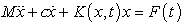(1)

where,and is the force vector of externally applied torques . Also x,y represents translations.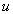is the rotational deflection (rotation in radians times the gear base radii or radius to the planet centre’s for the carrier) , and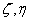are planet radial and tangential deflections;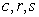represent the carrier, ring and sun, respectively , and the subscript fromdesignate the planet. The total number of degrees of freedom is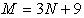. The damping matrixis obtained from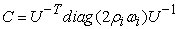, where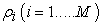is modal damping ratios approximating the material and bearing damping used in the finite element model, and the natural frequencies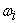and orthonormalized modal matrix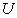are from the time-invariant system with average mesh stiffness. The inertias, gear geometry parameters, natural frequencies, and damping ratios of the various systems analyzed in this paper are taken as per reference . The nonlinearity from tooth contact loss is incorporated into the stiffness matrix through the sun planet and ring planet mesh stiffness’s as shown in Equation (2);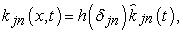(2)

where,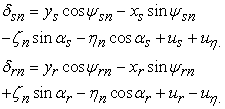where,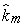is the linear, periodically varying mesh stiffness,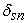andare the compressive deflections in the sun planet and ring planet mesh springs,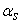andare the sun planet and ring planet operating pressure angles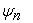is the circumferential position of planet n around the sun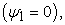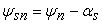and. Mesh phase relations are enforced according to Parker and Lin . A purely rotational model can be reduced from the above rotational translational system by removing the translations of the components. The rotational model allows mesh model verification isolated from the effects of bearing deflections. The equation of motion for a rotational model with N planets is [15, 16].(3)

Where,The inertia matrix,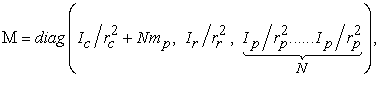(p represent planet gear) are the moments of inertia,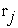is the base radii of the gears or radius of the carrier, mp is the mass of planet. The damping matrix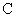is obtained as for a rotational-translational model. The stiffness matrix,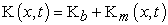whereis the torsional bearing stiffness , and the mesh stiffness matrix Km from Ref.[27, 28] is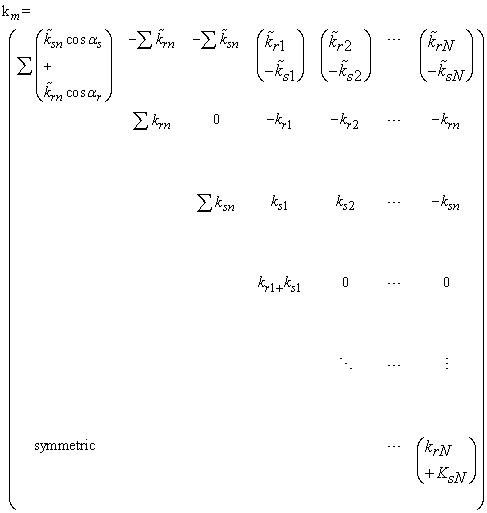(4)

where ,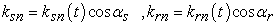Note in prior studies [10, 16] the factors of cosand cos,in their rotational model stiffness matrixes were mistakenly omitted. Nonlinearity from tooth contact loss is introduced into the sun planet and ring planet mesh stiffness’s based upon whether the mesh springs are in compression or not.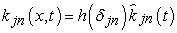(5)

Where,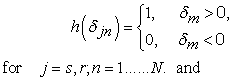(6)
3.2. Finite Element Model

To perform finite element analysis Ansys-14.5 software for gear dynamics is used to model the planetary gears. The finite element model does not require any external specification of the periodically varying mesh stiffness or static transmission error to excite the dynamics. The only inputs, in addition to the gear geometry and material properties, are the input torque and the speed of the gears. Mesh stiffness variation due to the change in the number of teeth in contact, corner contact due to the elastic deformations of the gear teeth, tooth contact loss, and gear body elastic compliance are all intrinsically modeled in the finite element model. Transmission error is a computed output; there is no need to invoke approximations with static transmission error as an excitation. This model significantly reduces the assumptions needed to model the complex dynamic mesh forces. Reference frames are attached to each component, and these rotate according to nominal trajectories from the rigid body kinematics of planetary gears. The calculated rotational and translational component vibrations are the deflections of the bodies from these nominal kinematic positions (i.e., dynamic transmission error and translational motions on bearings). Bearings are modeled with 3×3 stiffness matrices. Viscous bearing damping is included by a 3×3 damping matrix between the connecting bodies.

Small values of material damping coefficients α and β are introduced in the finite element models to remove numerical instabilities in the solution method. The Rayleigh damping model used here assumes that the damping of a finite element is proportional to the mass (Mfe) and stiffness (Kfe) of the element, i.e., Cfe= α Mfe+ β Kfe. The viscous bearing damping and the material damping coefficients are selected such that the damping ratios at the vibration modes of interest are less than 3%. These values are comparable to the 1% value that Blankenship and Kahraman  estimated for their experiments on a spur gear pair. The finite element model of an example system with three planets is shown in Figure 3(b). The carrier (not shown) is modeled as a lumped inertia. The gear geometry data is given in Table 2.1 -Table 2.8. All the planetary gear configurations analyzed in this study have the same gears and carrier. The exterior circle of the ring gear and the interior bores of the sun and planet gears are constrained to remain circular. In some applications, the gear bodies, especially the ring gear, may deform elastically into non-circular shapes, requiring an extension of the present analytical model. The outer ring gear circle is rigidly fixed in all systems considered. The constant input torque 2kNm is applied at the sun gear as shown in Figure 4. The carrier rotational vibration is constrained to zero, i.e., the carrier always rotates according to its nominal kinematic position, which removes the rigid body mode.Download asVeiw figureFigures index
Figure 4. PGT with boundary conditions

3.2.1. Set up Finite Element Model

A 3D entity model is imported into ANSYS Workbench, choosing materials for parts, giving material constants such as elasticity modulus, Poisson ratio, and density parameters, and meshing the entity model with refined grids for gear teeth parts, nevertheless relatively larger meshing for the rest of the parts aiming at expediting the solving effort. After partitioning, there are total 49947 nodes and 8043 element/units. See the Figure 3(b) for developed finite element model.

3.2.2 Analysis of the Load Sharing RatioDownload asVeiw figureFigures index
Figure 5. PGT with deformationDownload asVeiw figureFigures index
Figure 6. PGT with induced equivalent stress

### 4. Results and Discussion

This study has been carried out to evaluate the deformation and von-mises stress induced in the planetary gear-train by analytical and simulation approach using commercial software ANSYS 14.5. From analytical analysis it is seen that the total deformation induced in considered PGT as shown in Figure 3(b) was obtained to be 0.47631mm. Figure 5 shows the simulated deformed shape of PGT under consideration. Figure 5 shows the simulated maximum value of deformation induced in considered PGT to be 0.37631mm. After comparing the analytical and simulated values of induced deformation, it is found that the results are in good agreement. Again finite element analysis is performed to find out the minimum and maximum amount of stress in considered PGT. Initially, it was carried out for the existing model of the planet carrier, sun & planet teeth meshing, planet & ring gear teeth meshing of planetary gear train. In the considered PGT as shown in Figure 6, the minimum value of obtained induced equivalent stress is 1.9449e-015 MPa and the maximum value of obtained induced equivalent stress is 176.46 MPa. Again it is seen that the simulated values of equivalent stresses are in good agreement with design stress.

### 5. Conclusion

The primary objective of this work was to perform dynamic analysis of planetary gear train to study the effect of deflection and stresses to check the feasibility of considered PGT in earlier work as in Ref.. This objective was achieved with the help extensive analytical work and simulation work (finite element analysis) by using Ansys14.5. In this paper two independent models: one a lumped-parameter mathematical model and the other a finite element model, with different formulations and different mesh modeling assumptions are used to analyze the nonlinear dynamics of planetary gears. The main conclusions are: (1) there is strong agreement between the analytical and finite element model’s responses across a range of complicated nonlinear behaviors for planetary gear train; this confirms the validity of the lumped-parameter model for predicting planetary gear dynamics, (2) the simulated values of deflection and stresses are in good agreement with analytically calculated values.

### References

  S. H. Gawande , S. N. Shaikh, “Experimental Investigations of Noise Control in Planetary Gear Set by Phasing”., Journal of Engineering, 2014, 1-11, 2014.In article View Article  A. Kahraman , G.W. Blankenship, “Experiments on nonlinear dynamic behavior of an oscillator with clearance and periodically time varying parameters”. Journal of Applied Mechanics, 64 (1), 217-226, 1997.In article View Article  G.W. Blankenship, A. Kahraman, “Steady state forced response of a mechanical oscillator with combined parametric excitation and clearance type non-linearity”. Journal of Sound and Vibration, 185 (5), 743-765, 1995.In article View Article  M. Botman, “Vibration measurements on planetary gears of aircraft turbine engines”, Journal of Aircraft,17(5), 351-357, 1980.In article View Article  F. Cunliffe, J. D. Smith, D.B. Welbourn, “Dynamic tooth loads in epicyclic gears”. , 96(2), 578-584, 1974.In article View Article  M. Botman, “Epicyclic gear vibrations”. , 98 (3), 811-815, 1976.In article View Article  T. Hidaka, Y. Terauchi, “Dynamic behaviour of planetary gear (1st report: load distribution in planetary gear)”. Bulletin of the JSME Japan Society of Mechanical Engineers, 19(132), 690-698, 1976.In article View Article  T. Hidaka, Y. Terauchi, K. Ishioka, “Dynamic behaviour of planetary gear (2nd report: displacement of sun gear and ring gear)”. Bulletin of the JSME-Japan Society of Mechanical Engineers, 19 (138), 1563-1570, 1976.In article View Article  A. Kahraman, “Planetary gear train dynamics”. Journal of Mechanical Design, 116 (3), 713-720, 1994.In article View Article  A. Kahraman, “Natural-modes of planetary gear trains”. Journal of Sound and Vibration, 173 (1), 125-130, 1994.In article View Article  A. Kahraman, “Load sharing characteristics of planetary transmissions”. Mechanism and Machine Theory, 29(8), 1151-1165, 1994.In article View Article  J. Lin, R.G. Parker, “Analytical characterization of the unique properties of planetary gear free vibration”. Journal of Vibration and Acoustics, 121(3), 316-321, 1999.In article View Article  J. Lin, R.G. Parker, “Structured vibration characteristics of planetary gears with unequally spaced planets”. Journal of Sound and Vibration, 233(50), 921-928, 2000.In article View Article  J. Lin, R.G. Parker, “Sensitivity of planetary gear natural frequencies and vibration modes to model parameters”. Journal of Sound and Vibration, 228 (1), 109-128, 1999.In article View Article  J. Lin, R.G. Parker, “Natural frequency veering in planetary gears”. Mechanics of Structures and Machines, 29(4), 411-429, 2001.In article View Article  J. Lin, R.G. Parker, “Planetary gear parametric instability caused by mesh stiffness variation”. Journal of Sound and Vibration, 249 (1), 129-145, 2002.In article View Article  A. Kahraman, G.W. Blankenship, “Planet mesh phasing in epicyclic gear sets”. International Gearing Conference, Newcastle, Wash, USA, 1994.In article  R.G. Parker, “A physical explanation for the effectiveness of planet phasing to suppress planetary gear vibration”. Journal of Sound and Vibration, 236(4), 561-573, 2000.In article View Article  R.G. Parker, S.M. Vijayakar, T. Imajo, “Non-linear dynamic response of a spur gear pair: modeling and experimental comparisons”. Journal of Sound and Vibration, 237(3), 435-455, 2000.In article View Article  R.G. Parker, V. Agashe, S.M. Vijayakar, “Dynamic response of a planetary gear system using a finite element/contact mechanics model”. Journal of Mechanical Design, 122(3), 304-310, 2000.In article View Article  A. Kahraman, S.M. Vijayakar, “Effect of internal gear flexibility on the quasi-static behavior of a planetary gear set”. Journal of Mechanical Design,123(3), 408-415, 2001.In article View Article  A. Kahraman, A.A. Kharazi, M. Umrani, “A deformable body dynamic analysis of planetary gears with thin rims”. Journal of Sound and Vibration, 262(3), 752-768, 2003.In article View Article  C. Yuksel, A. Kahraman, “Dynamic tooth loads of planetary gears sets having tooth profile wear”. Mechanisms and Machine Theory, 39 (7), 695-715, 2004.In article View Article  R. J. Drago, “How to design quiet transmissions”. Machine Design, 52(28), 175-181, 1980.In article  H.N.Özguven, D.R. Houser, “Mathematical models used in gear dynamics – A review”. Journal of sound and vibration, 121 (3), 383-411, 1988.In article View Article  S.H.Gawande, S.N.Shaikh, R.N.Yerrawar, and K.A.Mahajan, “Noise Level Reduction in Planetary Gear Set”. Journal of Mechanical Design & Vibration, 2 (3), 60-62, 2014.In article  V.K. Ambarisha, R.G. Parker, “Suppression of planet mode response in planetary gear dynamics through mesh phasing”. Journal of Vibration and Acoustics, 128(2), 133-142, 2006.In article View Article  J. Wang, Y. Wang, and Z. Huo, “Finite Element Residual Stress Analysis of Planetary Gear Tooth”. Advances in Mechanical Engineering, 2013 ,1-12, 2014.In article